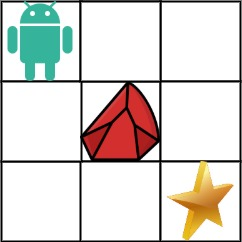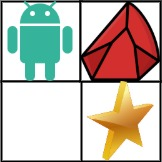# 63. Unique Paths II

Medium
You are given an `m x n` integer array `grid`. There is a robot initially located at the top-left corner (i.e., `grid`). The robot tries to move to the bottom-right corner (i.e., `grid[m - 1][n - 1]`). The robot can only move either down or right at any point in time.
An obstacle and space are marked as `1` or `0` respectively in `grid`. A path that the robot takes cannot include any square that is an obstacle.
Return the number of possible unique paths that the robot can take to reach the bottom-right corner.
The testcases are generated so that the answer will be less than or equal to `2 * 109`.
Example 1:Input: obstacleGrid = [[0,0,0],[0,1,0],[0,0,0]]
Output:
2
Explanation:
There is one obstacle in the middle of the 3x3 grid above.
There are two ways to reach the bottom-right corner:
1. Right -> Right -> Down -> Down
2. Down -> Down -> Right -> Right
Example 2:Input: obstacleGrid = [[0,1],[0,0]]
Output:
1
Constraints:
• `m == obstacleGrid.length`
• `n == obstacleGrid[i].length`
• `1 <= m, n <= 100`
• `obstacleGrid[i][j]` is `0` or `1`.

### 解題

func uniquePathsWithObstacles(obstacleGrid [][]int) int {
dp := obstacleGrid
if obstacleGrid == 0 {
dp = 1
} else {
dp = 0
}
for i := 1; i < len(dp); i++ {
if obstacleGrid[i] == 0 {
dp[i] = dp[i - 1]
} else {
dp[i] = 0
}
}
for i := 1; i < len(dp); i++ {
if obstacleGrid[i] == 0 {
dp[i] = dp[i - 1]
} else {
dp[i] = 0
}
}
for i := 1; i < len(dp); i++ {
for j := 1; j < len(dp); j++ {
if obstacleGrid[i][j] == 0 {
dp[i][j] = dp[i - 1][j] + dp[i][j - 1]
} else {
dp[i][j] = 0
}
}
}
return dp[len(dp) - 1][len(dp) - 1]
}